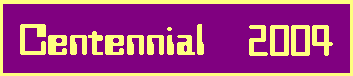# 2-Stage Metabelian 3-Groups

## 3.A. All known sextic S3 examples:

In the following diagrams, we give all 2-stage metabelian 3-groups G = G(K*1|k0) that occured for sextic S3 fields K over the quadratic cyclotomic field k0 = Q((-3)1/2) having 2-prime conductors f = 32*q with a prime q = -1(mod 9) . We denote by G = G1 > G2 > ... > Gi > ... > Gm = 1 the descending central series of class m - 1 of G with Gi+1 = [Gi,G]. In particular, G2 = [G,G] is the commutator subgroup G' of G.
The relative 3-genus field K* = (K|k0)* of K over k0 is the compositum K* = k*k~ of k = k0(q1/3) and k~ = k0(31/3), the cubic Kummer extensions of k0 with the two primeconductors q and 9 dividing f. Hence K* is a bicyclic bicubic relative extension of k0 that contains yet two other cubic Kummer extensions K = k0((3q)1/3) and K~ = k0((9q)1/3) of k0 with conductor f.

We gratefully acknowledge that Karim Belabas has computed the structure of G2 = Syl3C(K*) with the aid of PARI.
Group of maximal class
(e = 2)
G = G(5)(alpha,beta,gamma)
in ZEF 2a(5,5)
with (alpha,beta,gamma) = (0,0,0),
of class 4 and of order 35 = 243
 K*1 = K~1 | F4 | K1 | K* / / \ \ k K K~ k~ \ \ / / k0
 G5 = 1 | G4 = (3) | G3 = (3,3) | G2 = (32,3) / / \ \ h H H~ h~ \ \ / / G
Minimal occurrence:
f = 153 = 32*17
3-class numbers of K and K~:
(9,81)
principal factorization types of K and K~:
(BETA,GAMMA) .
Group of maximal class
(e = 2)
G = G(4)(alpha,beta,gamma)
in ZEF 2a(4,4)
with (alpha,beta,gamma) = (1,1,0),
of class 3 and of order 34 = 81
 K*1 | K1 = K~1 | K* / / \ \ k K K~ k~ \ \ / / k0
 G4 = 1 | G3 = (3) | G2 = (3,3) / / \ \ h H H~ h~ \ \ / / G
Minimal occurrence:
f = 801 = 32*89
3-class numbers of K and K~:
(9,9)
principal factorization types of K and K~:
(BETA,BETA) .
Group of lower than second maximal class
(e = 4)
G = G(6,8)((alpha,beta,gamma,delta),rho)
in ZEF(6,8)
of class 5 and of order 38 = 6561
 K*1 | F5 || K1 = K~1 || F3 | K* / / \ \ k K K~ k~ \ \ / / k0
 G6 = 1 | G5 = (3) || G4 = (3,3,3) || G3 = (32,3,3,3) | G2 = (32,32,3,3) / / \ \ h H H~ h~ \ \ / / G
Minimal occurrence:
f = 2421 = 32*269
3-class numbers of K and K~:
(81,81)
principal factorization types of K and K~:
(BETA,BETA) .
Group of lower than second maximal class
(e = 4)
G = G(7,9)((alpha,beta,gamma,delta),rho)
in ZEF2(7,9)
of class 6 and of order 39 = 19683
 K*1 | F6 | K~1 || K1 || F3 | K* / / \ \ k K K~ k~ \ \ / / k0
 G7 = 1 | G6 = (3) | G5 = (3,3) || G4 = (32,3,3) || G3 = (32,32,3,3) | G2 = (33,32,3,3) / / \ \ h H H~ h~ \ \ / / G
Minimal occurrence:
f = 7443 = 32*827
3-class numbers of K and K~:
(81,729)
principal factorization types of K and K~:
(BETA,GAMMA) .
Group of lower than second maximal class
(e = 4)
G = G(9,11)((alpha,beta,gamma,delta),rho)
in ZEF2(9,11)
of class 8 and of order 311 = 177147
 K*1 | F8 | K~1 | F6 | F5 || K1 || F3 | K* / / \ \ k K K~ k~ \ \ / / k0
 G9 = 1 | G8 = (3) | G7 = (3,3) | G6 = (32,3) | G5 = (32,32) || G4 = (33,32,3) || G3 = (33,33,3,3) | G2 = (34,33,3,3) / / \ \ h H H~ h~ \ \ / / G
Minimal occurrence:
f = 9873 = 32*1097
3-class numbers of K and K~:
(81,6561)
principal factorization types of K and K~:
(BETA,GAMMA) .
 References:  Brigitte Nebelung, Klassifikation metabelscher 3-Gruppen mit Faktorkommutatorgruppe vom Typ (3,3) und Anwendung auf das Kapitulationsproblem, Inauguraldissertation, Köln, 1989  Aïssa Derhem, Retour sur la thèse de Moulay Chrif Ismaïli, Casablanca, 2003  Daniel C. Mayer, Class Numbers and Principal Factorization Types of Multiplets of Pure Cubic Fields Q( R1/3 ) with R < 106, Univ. Graz, Computer Centre, 2003

 <| Navigation Center <| <| Back to Algebra <|